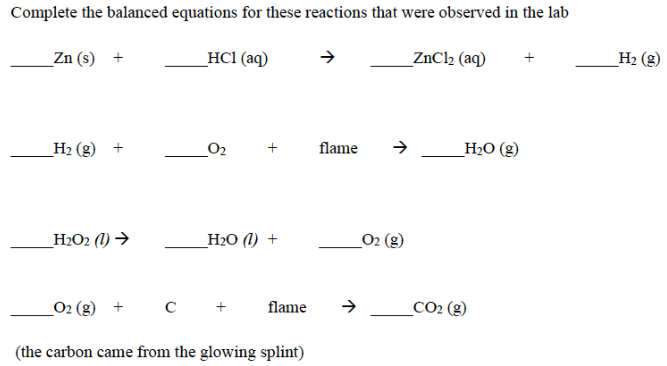# Problem: Complete the balanced equations for these reactions that were observed in the lab.___Zn (s) + ___ HCl (aq) → ZnCl2 (aq) + ___ H2 (g) ___ H2 (g) + ___ O2 + flame  →  ___ H2O (g) ___ H2O2 (l)  → ___ H2O (l) + ___ O2 (g) ___ O2 (g) + C + flame → ___ CO2 (g) (the carbon came from the glowing splint)

###### FREE Expert Solution
80% (257 ratings)###### Problem Details

Complete the balanced equations for these reactions that were observed in the lab.

___Zn (s) + ___ HCl (aq) → ZnCl2 (aq) + ___ H2 (g)

___ H2 (g) + ___ O+ flame  →  ___ H2O (g)

___ H2O2 (l)  → ___ H2O (l) + ___ O2 (g)

___ O2 (g) + C + flame → ___ CO2 (g)

(the carbon came from the glowing splint)